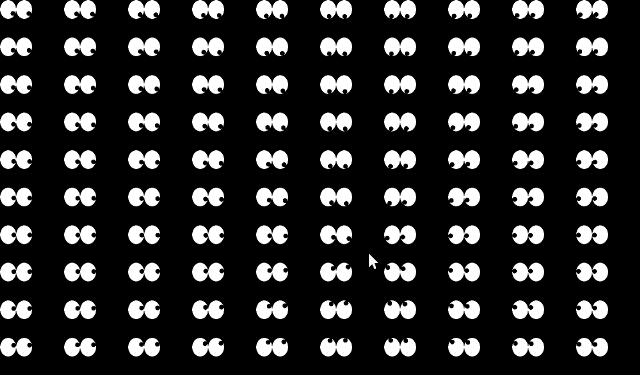## feeling watched in openframeworks

I wrote another little app in openframeworks today to test of006 which came out a couple of days ago.

i always liked those little xeyes apps (and all its windows, mac, whatever clones) so i took it to the extrem and made a LOT of them - feel beeing watched :-)testApp.h:

``````#ifndef _TEST_APP
#define _TEST_APP

#include "ofMain.h"
class testApp : public ofBaseApp{
public:
void setup();
void draw();

private:
void eye( int x, int y, int w, int h, int lookAtX, int lookAtY );
};
#endif
``````

testApp.cpp:

``````#include "testApp.h"

void testApp::setup() {
ofEnableSmoothing();
}

void testApp::draw(){
ofBackground(0.0,0.0,0.0);
for ( int x = 0; x < 10; x ++ ) {
for ( int y = 0; y < 10; y ++ ) {
eye( x * ofGetWidth()/10, y * ofGetHeight()/10, ofGetWidth() / 20, ofGetHeight()/20, mouseX, mouseY );
}
}
}

void testApp::eye( int x, int y, int w, int h, int lookAtX, int lookAtY ) {
float er = min( w, h ) / 4;

float d1 = ( y + h/2 - (float)lookAtY )/( x + w/4 - (float)lookAtX);
float a1 = atan( d1 ) + ( lookAtX < x +w/4 ? PI : 0 );
float d2 = ( y + h/2 - (float)lookAtY )/( x + 3*w/4 - (float)lookAtX);
float a2 = atan( d2 ) + ( lookAtX < x + 3*w/4 ? PI : 0 );

ofSetColor(255,255,255);
ofEllipse( x + w/4, y + h/2, w/2, h);
ofEllipse( x + 3*w/4,  y + h/2, w/2, h);

ofSetColor( 0,0,0);
ofEllipse( x + w/4 + cos( a1 ) * (w/4 - er/2), y + h/2 + sin( a1 ) * (h/2 - er/2), er, er );
ofEllipse( x + 3*w/4 + cos( a2 ) * (w/4 - er/2), y + h/2 + sin( a2 ) * (h/2 - er/2), er, er );
}
``````